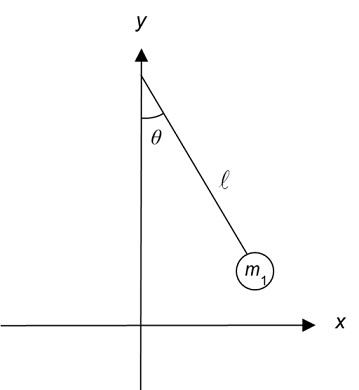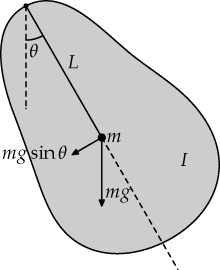# Difference Between Simple Pendulum and Compound Pendulum

## Main Difference – Simple Pendulum vs. Compound Pendulum

pendulum consists of an object suspended along an axis so that it is able to move back and forth freely. Depending on the shape of the pendulum, a pendulum could be classified as a simple pendulum or a compound pendulum (physical pendulum). The main difference between simple pendulum and compound pendulum is that, in a simple pendulum, the distance between the centre of gravity of the suspended body and the axis of suspension is large compared to the dimensions of the suspended body whereas, in a compound pendulum, the dimensions of the suspended body are comparable to the distance between the body’s centre of gravity and the axis of suspension. There is no clear-cut definition to differentiate between a simple pendulum and a compound pendulum, however, so this is a rather qualitative distinction.

## What is a Simple Pendulum

As mentioned earlier, in a simple pendulum the dimensions of the object in suspension is significantly smaller than the distance from the centre of gravity of the object to the axis of suspension. This allows us to treat the mass as though it were a single point. We also assume the “string” suspending the body is inelastic and massless. The figure below illustrates a simple pendulum:A simple pendulum

We take the length$l$ to be the distance from the axis of suspension to the centre of gravity of the body (the body in motion in a simple pendulum is often called the “bob”). Then, if we let the bob to oscillate back-and-forth with a small angle$\theta$, then we can show that the body will oscillate with a period$T$ given by:$T=2\pi \sqrt{\frac{l}{g}}$

Note: the period is the time that the body takes to complete one full swing.$g$ is the acceleration due to gravity, with$g=9.81\mathrm{\:m\:s^{-2}}$.

## What is a Compound Pendulum

In a compound pendulum, the distance between the centre of gravity of the swinging body and the axis of suspension is comparable to the dimensions of the body. The figure below illustrates a compound pendulum:A compound pendulum

The body is suspended at the top. Here, the point marked with a dot is the centre of gravity and the length$L$ is the distance between the centre of gravity and the axis of suspension. For a compound pendulum oscillating with a small angle, it can be shown that the period$T$ with which it oscillates is given by:$T=2\pi \sqrt{\frac{I}{mgL}}$

Here,$I$ is the moment of inertia of the body about the axis of rotation.$m$ refers to the mass of the body.

## Difference Between Simple Pendulum and Compound Pendulum

### Description

In a simple pendulum, the oscillating mass has dimensions much smaller than the distance between the axis of suspension and the centre of gravity.

In a compound pendulum, the oscillating mass has dimensions comparable to the distance between the axis of suspension and its centre of gravity.

### Determination of the Period

In a simple pendulum, the only physical property of the pendulum that affect its period is its length. The mass of the object does not affect the period.

In a compound pendulum, the object’s mass and how that mass is distributed both play a role in determining the period.

Image Courtesy

“Simple pendulum.” by F=q(E+v^B) (Own work) [CC BY-SA 3.0], via Wikimedia Commons

“Physical pendulum (labeled diagram).” by Malak, Michael (Own work) [Public Domain], via Wikimedia Commons